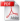# McWhorter and Sunada Exact Solution

This is the online implementation of the 1D integral solution of two-phase flow in porous media by McWhorter and Sunada (1990) and Fučík et al. (2007). Please, cite our work (see references below).

## Parameters

Model selection

Model selection

 Material properties $\phi$  porosity $K$ [m$^2$] intrinsic permeability $S_{wr}$  residual saturation of the wetting phase $S_{nr}$  residual saturation of the non-wetting phase Fluid properties $\mu_w$ [kg/m/s] dynamic viscosity of the wetting phase $\mu_n$ [kg/m/s] dynamic viscosity of the non-wetting phase Brooks and Corey model parameters $p_d$ [Pa] Brooks and Corey model parameter: entry pressure $\lambda$  Brooks and Corey model parameter: pore size distribution index van Genuchten mode parameters $\alpha$ [1/Pa] van Genuchten model parameter $m$  van Genuchten model parameter $n$  van Genuchten model parameter Problem parameters $S_0$  injection boundary saturation $S_i$  initial saturation $R$  flux parameter Computation parameters $t$ [s] the solution will be plotted and exported at this time nodes length of the discrete vector max_iter maximum number of iterations $\epsilon$ stopping criterion of the iterations

Computational method selection

## References

• link R. Fučík, T. H. Illangasekare, and M. Beneš Multidimensional self-similar analytical solutions of two-phase flow in porous media, Advances in Water Resources, Volume 90, April 2016, Pages 51–56 doi.org/10.1016/j.advwatres.2016.02.007
•pdf R.Fučík, J. Mikyška, T. H. Illangasekare and M. Beneš: Semianalytical Solution for Two-Phase flow in Porous Media with a Discontinuity Vadose Zone Journal 2008 vol. 7, no. 3: pages 1001-1009
•pdf R.Fučík, J. Mikyška, T. H. Illangasekare and M. Beneš: An Improved Semi-Analytical Solution for Verification of Numerical Models of Two-Phase Flow in Porous Media Vadose Zone Journal 2007 no. 6: pages 93-104pdf (1,01 MB) corrected version